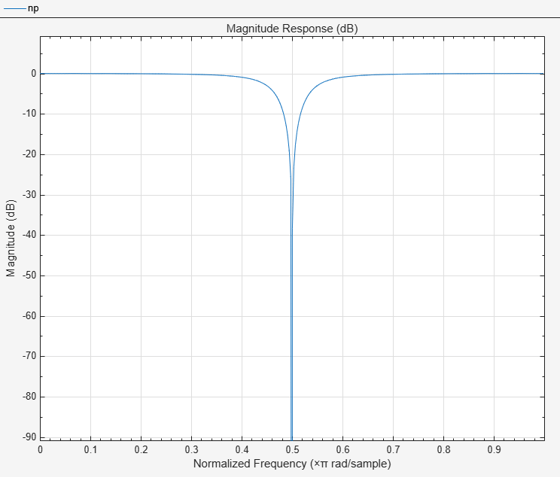getCenterFrequency

Get center frequency

Description

example

CF = getCenterFrequency(npFilter) returns the center frequency of the notch peak filter. If the Specification property is set to 'Coefficients', the center frequency is determined from the CenterFrequencyCoefficient value and the sample rate.

Examples

collapse all

Create a dsp.NotchPeakFilter object with the Specification property set to 'Coefficients'.

np = dsp.NotchPeakFilter('Specification','Coefficients')
np =
dsp.NotchPeakFilter with properties:

Specification: 'Coefficients'
BandwidthCoefficient: 0.7265
CenterFrequencyCoefficient: 0
SampleRate: 44100

Determine the center frequency of the notch peak filter using the getCenterFrequency function. When the Specification is set to 'Coefficients', the center frequency is determined from the CenterFrequencyCoefficient value and the sample rate.

getCenterFrequency(np)
ans = 11025

Visualize the filter response using fvtool.

fvtool(np)Input Arguments

collapse all

Notch peak filter whose center frequency is measured, specified as dsp.NotchPeakFilter object.

Output Arguments

collapse all

Center frequency of the filter, returned as a scalar.

Data Types: double

Objects

Introduced in R2014a

DSP System Toolbox Documentation평가판 신청